## TensorFlow 2.0 实现线性回归

2629 字 · 3671 阅读 · 2019 年 04 月 02 日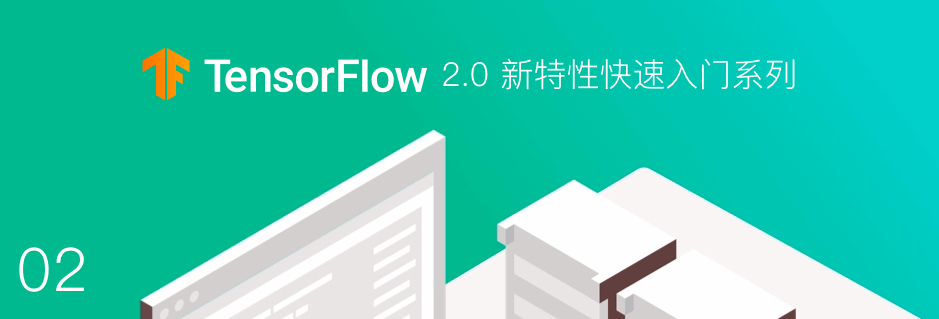### 低阶 API 实现

import matplotlib.pyplot as plt
import tensorflow as tf
%matplotlib inline

TRUE_W = 3.0
TRUE_b = 2.0
NUM_SAMPLES = 100

# 初始化随机数据
X = tf.random.normal(shape=[NUM_SAMPLES, 1]).numpy()
noise = tf.random.normal(shape=[NUM_SAMPLES, 1]).numpy()
y = X * TRUE_W + TRUE_b + noise  # 添加噪声

plt.scatter(X, y)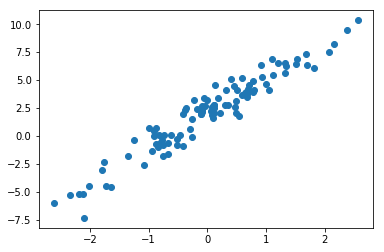$$f(w, b, x) = w*x + b$$

class Model(object):
def __init__(self):
self.W = tf.Variable(tf.random.uniform())  # 随机初始化参数
self.b = tf.Variable(tf.random.uniform())

def __call__(self, x):
return self.W * x + self.b  # w*x + b


model = Model()  # 实例化模型

plt.scatter(X, y)
plt.plot(X, model(X), c='r')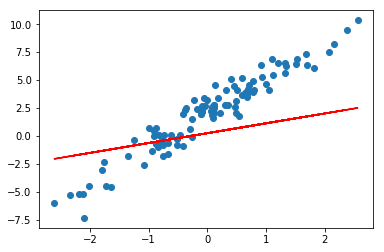$${\rm Loss}(w, b, x, y) = \sum_{i=1}^N (f(w, b, x_i) - y_i)^2$$

def loss_fn(model, x, y):
y_ = model(x)
return tf.reduce_mean(tf.square(y_ - y))TensorFlow 2.0 中的 Eager Execution 提供了 tf.GradientTape 用于追踪梯度。所以，下面我们就实现梯度下降法的迭代更新过程。

EPOCHS = 10
LEARNING_RATE = 0.1

for epoch in range(EPOCHS):  # 迭代次数
with tf.GradientTape() as tape:  # 追踪梯度
loss = loss_fn(model, X, y)  # 计算损失
dW, db = tape.gradient(loss, [model.W, model.b])  # 计算梯度
model.W.assign_sub(LEARNING_RATE * dW)  # 更新梯度
model.b.assign_sub(LEARNING_RATE * db)
# 输出计算过程
print('Epoch [{}/{}], loss [{:.3f}], W/b [{:.3f}/{:.3f}]'.format(epoch, EPOCHS, loss,
float(model.W.numpy()),
float(model.b.numpy())))

    Epoch [0/10], loss [9.949], W/b [1.395/0.608]
Epoch [1/10], loss [6.420], W/b [1.791/0.899]
Epoch [2/10], loss [4.270], W/b [2.097/1.132]
Epoch [3/10], loss [2.957], W/b [2.332/1.318]
Epoch [4/10], loss [2.156], W/b [2.514/1.466]
Epoch [5/10], loss [1.665], W/b [2.653/1.585]
Epoch [6/10], loss [1.365], W/b [2.761/1.680]
Epoch [7/10], loss [1.180], W/b [2.845/1.756]
Epoch [8/10], loss [1.067], W/b [2.909/1.817]
Epoch [9/10], loss [0.998], W/b [2.958/1.865]

plt.scatter(X, y)
plt.plot(X, model(X), c='r')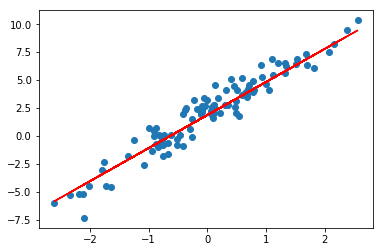### 高阶 API 实现

TensorFlow 2.0 中提供了大量的高阶 API 帮助我们快速构建所需模型，接下来，我们使用一些新的 API 来完成线性回归模型的构建。这里还是沿用上面提供的示例数据。

tf.keras 模块下提供的 tf.keras.layers.Dense 全连接层（线性层）实际上就是一个线性计算过程。所以，模型的定义部分我们就可以直接实例化一个全连接层即可。

model = tf.keras.layers.Dense(units=1)  # 实例化线性层


plt.scatter(X, y)
plt.plot(X, model(X), c='r')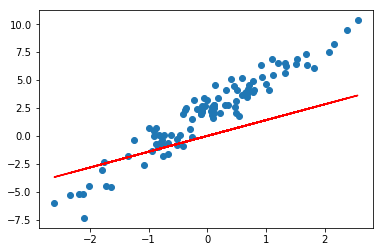model.variables

    [<tf.Variable 'dense/kernel:0' shape=(1, 1) dtype=float32, numpy=array([[1.4135073]], dtype=float32)>,
<tf.Variable 'dense/bias:0' shape=(1,) dtype=float32, numpy=array([0.], dtype=float32)>]

EPOCHS = 10
LEARNING_RATE = 0.002
for epoch in range(EPOCHS):  # 迭代次数
with tf.GradientTape() as tape:  # 追踪梯度
y_ = model(X)
loss = tf.reduce_sum(tf.keras.losses.mean_squared_error(y, y_))  # 计算损失

optimizer = tf.keras.optimizers.SGD(LEARNING_RATE)  # 随机梯度下降

print('Epoch [{}/{}], loss [{:.3f}]'.format(epoch, EPOCHS, loss))

    Epoch [0/10], loss [848.794]
Epoch [1/10], loss [339.506]
Epoch [2/10], loss [172.200]
Epoch [3/10], loss [116.692]
Epoch [4/10], loss [98.099]
Epoch [5/10], loss [91.816]
Epoch [6/10], loss [89.674]
Epoch [7/10], loss [88.938]
Epoch [8/10], loss [88.684]
Epoch [9/10], loss [88.596]

plt.scatter(X, y)
plt.plot(X, model(X), c='r')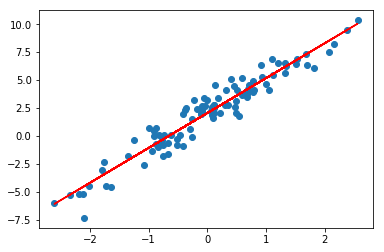### Keras 方式实现

model = tf.keras.Sequential()
model.summary()  # 查看模型结构

    Model: "sequential"
_________________________________________________________________
Layer (type)                 Output Shape              Param #
=================================================================
dense_1 (Dense)              (None, 1)                 2
=================================================================
Total params: 2
Trainable params: 2
Non-trainable params: 0
_________________________________________________________________

model.compile(optimizer='sgd', loss='mse')
model.fit(X, y, steps_per_epoch=1000)

    1000/1000 [==============================] - 1s 1ms/step - loss: 5.9901

steps_per_epoch 只的是在默认小批量为 32 的条件下，传入相应次数的小批量样本。最终绘制出迭代完成的拟合图像。

plt.scatter(X, y)
plt.plot(X, model(X), c='r')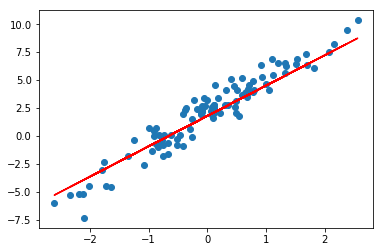### TensorFlow 1.x 实现

import tensorflow.compat.v1 as tf

tf.disable_v2_behavior() # 关闭 Eager Execution 特性


X_train = tf.placeholder(tf.float32)  # 定义占位符张量
y_train = tf.placeholder(tf.float32)
W = tf.Variable(tf.random.normal())  # 初始化参数
b = tf.Variable(tf.random.normal())

LEARNING_RATE = 0.001  # 学习率

y_train_ = W*X_train + b  # 线性函数
loss = tf.reduce_mean(tf.square(y_train_ - y_train))  # 平方损失函数
LEARNING_RATE).minimize(loss)  # 梯度下降优化损失函数

EPOCHS = 1000  # 迭代次数
with tf.Session() as sess: # 启动会话
tf.global_variables_initializer().run()  # 初始化全局变量
for epoch in range(EPOCHS):  # 迭代优化
sess.run(optimizer, feed_dict={X_train:X, y_train:y})
final_weight = sess.run(W)  # 最终参数
final_bias = sess.run(b)

print(final_weight, final_bias)

    [2.7856345] [1.6973654]

preds = final_weight * X + final_bias  # 计算预测值
plt.scatter(X, y)
plt.plot(X, preds, c='r')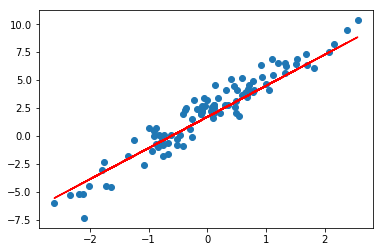#### 在线学习•